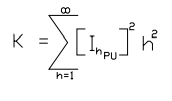Date:
Showing posts with label electrical harmonics. Show all posts
Showing posts with label electrical harmonics. Show all posts

## How to Determine the K- Rating of the Transformer?Power Transformer

The harmonic current can cause extra heating to transformers due to skin effect in coil conductors, extra eddy currents in core laminations, and excessive hysteresis (molecules rubbing against one another, similar to microwave oven operation).

After careful study using computer generated forecasting method, engineers developed a "k-rated transformer" that is designed to handle and mitigate the possible severity of the harmonics currents and its effect in the electrical system. A harmonic current content of k-4 is one that would cause heating equal to that which would have been caused by 1.140 times the load current had it all been fundamental (60-Hz) current.

The correct manner of determining the required k-rating of a transformer can be demonstrated by the following example:

Example: If the measured (or computer-forecast) current is as follows, what K-rating of transformer would be required to carry this load?

• True rms amperes = 73.3 amperes
• h1 = 52.45 amperes
• h3 = 42.27 amperes
• h5 = 24.97 amperes
• h7 = 9.44 amperes
• h9 = 3.72 amperes
• h11 = 5.51 amperes
• h13 = 4.77 amperesFormula

Consider IPU = Ih / IRMS

Using the above formula, we can come up with the following table of results.

From the result, the transformer should have a k-factor of 8.84 or above.

#### Harmonic Current Flow Thru Transformer

While most harmonic currents travel through transformers from the harmonic current–creating loads to the electrical power supply, some are captured within the transformer. The balanced triplen harmonic currents of the third, ninth, and fifteenth harmonics are captured within the delta winding of a transformer, where they simply circulate and heat the delta winding.

The only triplen harmonics that travel through a delta-wye, wye-delta, or wye-delta-wye transformer are unbalanced triplen harmonic currents.

Thus, the best way to eliminate the large portion of the harmonic currents is simply to insert a transformer with a delta coil winding into the power system to the load. Also, the alternative way to “cancel” the fifth and seventh harmonic currents from several loads is to connect some of them to delta-delta and some to delta-wye transformers, causing a 30° phase shift and a vector addition to almost zero of fifth and seventh harmonic currents.

This is the exact method used when installing a 12-pulse variable-speed drive (VSD) or variable frequency drive (VFD) instead of a 6-pulse drive, for the 12-pulse drive requires another transformer winding that is 30° phase-shifted from the first transformer’s secondary winding.